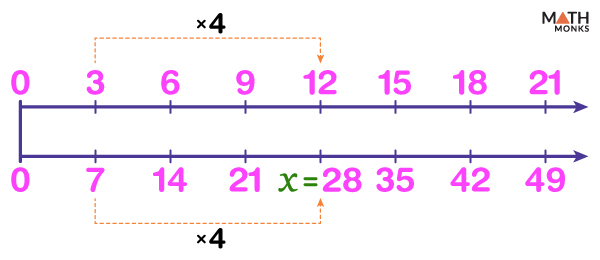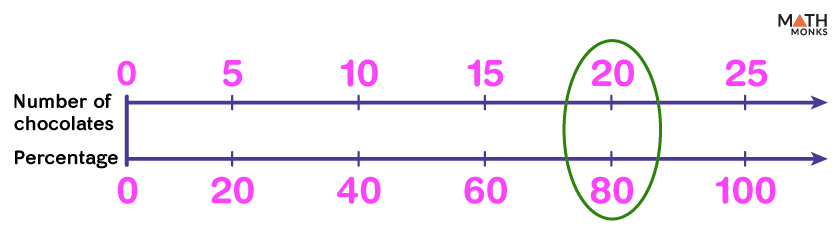# Double Number Line

A double number line is a pair of parallel, horizontal straight lines representing the corresponding values of two quantities with a constant ratio.

Let us assume Megan prepares chocolate milk by mixing 3 tablespoons of cocoa powder for every cup of milk.

Thus the simplest ratio between cups of milk and the amount of cocoa powder is 1:3. There are two ways to represent multiple batches of chocolate milk.

The diagram below shows one batch of the such recipe.

There is one large white square representing a cup of milk and 3 small brown squares representing 3 tablespoons of cocoa powder.

The above diagram is repeated the required number of times to get multiple batches of this recipe.

For example, 3 batches of the recipe are shown.

The best way to represent multiple batches of such data is by using a double number line.

For example, let us represent 6 batches of the above mix prepared by Megan.

Step 1: Two parallel lines with 6 evenly spaced tick marks are lined up at 0.

The top line is labeled as cups of milk, and the bottom line is tablespoons of cocoa powder.

The tick marks lining up with one another represent the values that correspond to one another. The 0th marking represents 0s in both the number lines.

Step 2: The first corresponding numbers next to 0s are 1 and 3, as the ratio of cups of milk to tablespoons of cocoa powder is 1:3.

Step 3: The subsequent values are filled by multiplying the original values by 2, 3, 4, 5, and 6 on each number line to get the equivalent ratios 2:6, 3:9, 4:12, 5:15, and 6:18.

Thus, we get the final double number line diagram by filling in all the above values.

Thus, using the above double number line, we can find the required quantity of cocoa powder, given the cups of milk, and vice versa.

For example, if asked how much cocoa powder Megan will require to prepare 5 cups of chocolate milk, we can quickly answer using the double number line. It is 15 tablespoons.

## Relation with Equivalent Ratio

A double number line thus establishes a relationship between two different quantities in the form of equivalent ratios.

The corresponding ratios, 1:3, 2:6, 3:9, 4:12, 5:15, and 6:18, are equivalent and can be presented as equivalent fractions. For examples, ${\dfrac{1}{3}}$ = ${\dfrac{5}{15}}$.

Let us consider another example.

Suppose there are 24 cookies in 6 boxes. We can calculate the number of cookies per box using a double number line diagram.

Here the ratio of the number of boxes to the number of cookies is given by 6:24, and the number of cookies per box is calculated by finding a ratio smaller than and equivalent to 6:24

Step 1: First, we draw a double number line diagram by plotting the original ratio of 6:24 to the right and leaving space for the smaller ratios.

Step 2: As the number of boxes in the original ratio is 6; both 6 and 24 are divided by 6 (the highest common factor of 6 and 24) to get the number of cookies per box. Thus, we get 1:4 as the ratio of boxes to cookies, which is the simplest form of the ratio.

Step 3: Similarly, multiplying 1 and 4 by 2, 3, 4,…, 6, we will obtain the corresponding values in each number line.

Thus, there are 4 cookies per box.Find the missing number in the given double number line.

Solution:Let the missing number on the second number line be x.
As we know, a double number line represent equivalent ratios; the value of 12:x will be equal to 3:7
i.e., ${\dfrac{3}{7}}$ = ${\dfrac{12}{x}}$
In the first number line, 12 is obtained by multiplying 3 by 4, and thus we will get the value of x by multiplying 7 by 4.
Thus, x = 7 × 4 = 28

## Solving Percentage Problems

A double number line also helps us to find the required percentage of an entire quantity.

Let us find 63 is 90% of what number.

Step 1: Let the required number be x, and the number in the first tick mark of the second number line be y.

Then, a double number line lined up at 0 is drawn. The numbers on the first and second lines indicate percentages from 0 to 100 and corresponding percentage values of ‘x’.

Thus, the pair of values (90, 63) and (100, x) are marked below.

Step 2: As 90% indicates the 9th part when 0 to 100 is divided into 10 equal parts, we divide the region from 0 to the last element in both the number lines into 10 equal parts.

Each part of the first number line represents 10%.

Step 3: Since a double number line represents equivalent ratios, the ratio in the 1st marking will equal that in the 9th. i.e., ${\dfrac{10}{y}}$ = ${\dfrac{90}{63}}$

90% is obtained by multiplying 10% by 9, so 63 is also obtained by multiplying y by 9

So, y = ${\dfrac{63}{9}}$ = 7

Step 4: As the quantities on the first number line are obtained by multiplying 10% by 1, 2, 3, 4,…,10; we get the corresponding numbers in the second line by multiplying 7 by 1, 2, 3, 4,….,10.

So the numbers on the bottom line are 7, 14, 21, 28, 35, 42, 49, 56, 63, and 70.

Thus we get x = 70.

So 63 is 90% of 70.

There were 25 chocolates in a box. Robert took 20 chocolates and distributed them among his friends. What percentage of chocolates was used by Robert?

Solution:To solve the problem, first, we draw a double number line diagram lined up at 0. The top line indicates the number of chocolates, and the bottom line shows the corresponding percentage of the total chocolates. Here a total of 25 chocolates correspond to 100%.

Now as ${\dfrac{20}{25}}$ = ${\dfrac{4}{5}}$, this marking denotes the 4th part starting from 0 on the first number line where the region from 0 to 25 is divided into 5 equal parts. So the numbers on the first line are the first five multiples of 5 (i.e., 5, 10, 15, 20, 25).

Thus in the bottom line, the region from 0 to 100% is also divided into 5 equal parts. Each part represents 20%, and the percentage values increase by 20% towards the right from 0.

Thus, the percentage corresponding to 20 chocolates is given by ${\dfrac{4}{5}th}$ part of 100%, i.e., 80%